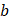# In the van der Waals equation a)is the volume occupied by the gas molecules b)is four times the volume occupied by the gas molecules c)is the correction factor for intermolecular attraction d) None of these

## Question ID - 102725 :- In the van der Waals equation a)is the volume occupied by the gas molecules b)is four times the volume occupied by the gas molecules c)is the correction factor for intermolecular attraction d) None of these

3537

(b)(co-volume)(Volume occupied by gaseous molecule)

Next Question :
 An ideal gas obeying kinetic theory of gases can be liquefied, if a) Its temperature is more than critical temperatureb) Its pressure is more than critical pressurec) Its pressure is more thanat a temperature less thand) It cannot be liquefied at any value ofand